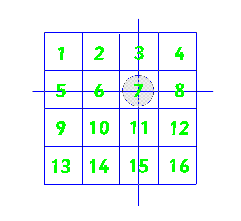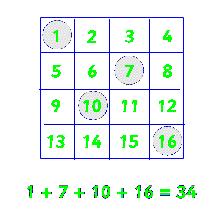#### You may also like### Summing Consecutive Numbers

15 = 7 + 8 and 10 = 1 + 2 + 3 + 4. Can you say which numbers can be expressed as the sum of two or more consecutive integers?### Fibs

The well known Fibonacci sequence is 1 ,1, 2, 3, 5, 8, 13, 21.... How many Fibonacci sequences can you find containing the number 196 as one of the terms?### Hallway Borders

What are the possible dimensions of a rectangular hallway if the number of tiles around the perimeter is exactly half the total number of tiles?

# Always the Same

##### Age 11 to 14Challenge Level

Arrange the numbers 1 to 16 into a 4 by 4 array.Choose a number and circle it. Cross out the numbers which are in the same row and column as your chosen number. Repeat this process twice more choosing from the remaining numbers. This should leave you with one number remaining, circle this one also.Add up your four circled numbers. Why do they always add up to 34 whatever way you choose your numbers?